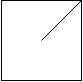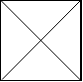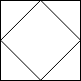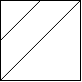# Posts tagged as “merge”

In a N x N grid composed of 1 x 1 squares, each 1 x 1 square consists of a /\, or blank space.  These characters divide the square into contiguous regions.

(Note that backslash characters are escaped, so a \ is represented as "\\".)

Return the number of regions.

Example 1:

Input:[  " /",  "/ "]Output: 2Explanation: The 2x2 grid is as follows:Example 2:

Input:[  " /",  "  "]Output: 1Explanation: The 2x2 grid is as follows:Example 3:

Input:[  "\/",  "/\"]Output: 4Explanation: (Recall that because \ characters are escaped, "\/" refers to \/, and "/\" refers to /\.)The 2x2 grid is as follows:Example 4:

Input:[  "/\",  "\/"]Output: 5Explanation: (Recall that because \ characters are escaped, "/\" refers to /\, and "\/" refers to \/.)The 2x2 grid is as follows:Example 5:

Input:[  "//",  "/ "]Output: 3Explanation: The 2x2 grid is as follows:Note:

1. 1 <= grid.length == grid.length <= 30
2. grid[i][j] is either '/''\', or ' '.

# Solution 1: Split grid into 4 triangles and Union Find Faces

Divide each grid into 4 triangles and union them if not split.
Time complexity: O(n^2*alphn(n^2))
Space complexity: O(n^2)

# Solution 3: Pixelation (Upscale 3 times)

Time complexity: O(n^2)
Space complexity: O(n^2)

# Problem

Merge k sorted linked lists and return it as one sorted list. Analyze and describe its complexity.

Example:

Input:
[
1->4->5,
1->3->4,
2->6
]
Output: 1->1->2->3->4->4->5->6

# Solution 1: Brute Force

Time complexity: O(nk)

Space complexity: O(1)

# Solution 2: Heap / Priority Queue

Time complexity: O(nlogk)

Space complexity: O(k)

# Problem

Given two sorted integer arrays nums1 and nums2, merge nums2 into nums1 as one sorted array.

Note:

• The number of elements initialized in nums1 and nums2 are m and n respectively.
• You may assume that nums1 has enough space (size that is greater or equal to m + n) to hold additional elements from nums2.

Example:

Input:
nums1 = [1,2,3,0,0,0], m = 3
nums2 = [2,5,6],       n = 3

Output: [1,2,2,3,5,6]


# Solution:

Fill nums1 from back to front

Time complexity: O(m + n)

Space complexity: O(1) in-place

# Problem

Given two binary trees and imagine that when you put one of them to cover the other, some nodes of the two trees are overlapped while the others are not.

You need to merge them into a new binary tree. The merge rule is that if two nodes overlap, then sum node values up as the new value of the merged node. Otherwise, the NOT null node will be used as the node of new tree.

Example 1:

Input:
Tree 1                     Tree 2
1                         2
/ \                       / \
3   2                     1   3
/                           \   \
5                             4   7
Output:
Merged tree:
3
/ \
4   5
/ \   \
5   4   7


Note: The merging process must start from the root nodes of both trees.

# Solution: Recursion

Reuse t1/t2

Create a copy

Problem:

Given a set of non-overlapping intervals, insert a new interval into the intervals (merge if necessary).

You may assume that the intervals were initially sorted according to their start times.

Example 1:
Given intervals [1,3],[6,9], insert and merge [2,5] in as [1,5],[6,9].

Example 2:
Given [1,2],[3,5],[6,7],[8,10],[12,16], insert and merge [4,9] in as [1,2],[3,10],[12,16].

This is because the new interval [4,9] overlaps with [3,5],[6,7],[8,10].

Idea:

Find the position of the new interval, insert it into the list and call MergeIntervals in LeetCode 56

Solution:

C++

Python

Solution 2:

C++

Python

Related problems:

Mission News Theme by Compete Themes.